# Principal Component Analysis for Numerals

3632 words (15 pages) Essay

22nd Jun 2018 Computer Science Reference this

Disclaimer: This work has been submitted by a university student. This is not an example of the work produced by our Essay Writing Service. You can view samples of our professional work here.

Any opinions, findings, conclusions or recommendations expressed in this material are those of the authors and do not necessarily reflect the views of UKEssays.com.

### Recognition of Off-Line Handwritten Telugu Numerals Based On Principal Component Analysis

• Manubolu Sreenivasulu, Prasenjit Kumar Das

### Abstract

India is a multi-lingual multi-language country but there is not much work towards off-line handwritten character recognition of Indian languages . In this paper we have proposed principal component analysis (PCA) for feature extraction and used Probabilistic Neural Network (PNN) based model for classification and recognition of off-line handwritten numerals of telugu script. Principal component analysis is one of the important method for identifying patterns in data and expressing the data to highlight their similarities and differences. In high dimensional data it is difficult to identify patterns, where the luxury of graphical representation is not available, principal component analysis is a good method for analysing data. The performance of the Probabilistic Neural Network classifier was computed in terms of training performance and classification accuracies. Probabilistic Neural Network gives fast and accurate classification and is a promising model for classification of the characters .

Keywords – PCA, PNN, Classification and Recognition, Handwritten Characters

## I. INTRODUCTION

Character recognition (CR) is the important area in image processing and pattern recognition fields. The various applications of character recognition are includes library automation, banks, defense organizations, reading aid for the blind, post offices, language processing and multi-media design. Hence the research in character recognition is very popular. To recognize Hand-Written Characters (HWC) is an easy task for humans, but for a computer it is an extremely difficult job. This is mainly due to the vast differences or the impreciseness associated with handwritten patterns written by different individuals. Machine recognition involves the ability of a computer to receive input from sources such as paper and other documents, photographs, touch screens and other devices, which is an ongoing research area. Handwritten character recognition (HWCR) can be divided into two categories, namely, Offline Handwritten Character recognition where the image is sensed “off-line” from a part of a document and “Online” Handwritten Character recognition where the movements of the pen/tip can be recorded “on-line” as used in the pen based computer screen systems. Off-line recognition usually requires imperfect pre-processing techniques prior to feature extraction and recognition stages .

If you need assistance with writing your essay, our professional essay writing service is here to help!

Telugu is the most popular script in India. It is the official language of the southern Indian state, Andhra Pradesh. The Telugu script is closely related to the Kannada script. Telugu is a syllabic language. Similar to most languages of India, each symbol in Telugu script represents a complete syllable. Officially, there are 10 numerals, 18 vowels, 36 consonants, and three dual symbols .

There are five major stages in the HCR problem: Image pre-processing, segmentation, feature extraction, training and recognition and post processing. Several works had been done on feature extraction for character recognition. The feature extraction method includes Template matching, Identification of similarities and differences, Histograms, Zoning, Graph description etc.

## II. DATA COLLECTION AND PREPROCESSING

Data collection for the experiment has been done from different individuals. Currently we are developing data set for Telugu. Earlier we had collected 250 Telugu numeral samples from 50 different writers. Writers were provided with a plain A4 sheet and each writer asked to write Telugu numerals from 0 to 9 at one time. Recently, we have again collected 150 Telugu numerals by 30 different writers. In this paper the data set size of 300 Telugu numerals is used. The database is totally unconstrained and has been created for validating the recognition system. The collected documents are scanned using the Canon-Lide scanner jet, which is usually a low noise and good quality image. The digitized images are stored as binary images in the BMP format. A sample of Telugu handwritten numerals from the data set is shown from figure 1.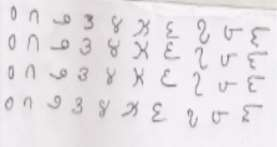Figure 1: Handwritten Telugu Numeral Samples

Pre-processing: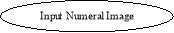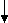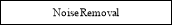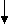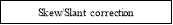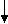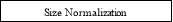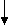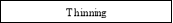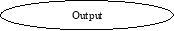Figure 2: Pre-processing

Pre-processing includes the steps that are necessary to bring the input data into an acceptable form for feature extraction. The initial data is depending on the data acquisition type, is subjected to a number of preliminary processing stages. The pre-processing stage involves noise reduction, slant correction, size normalization and thinning .

For noise removal we used median filter. For better understanding the function of median filter, we added the salt and pepper noise artificially and removing it using median filter.

In character classification/recognition correcting the skew (baseline deviation from the horizontal direction) and the slant (average near-vertical strokes deviation from the vertical direction) is an important pre-processing step. The slant and slope are introduced by writing styles. Both corrections can reduce handwritten word shape variability and help the later operations such as feature extraction, classification and recognition .

Normalization is required as the size of the numeral varies from person to person and even with the same person from time to time. Thinning provides a reduction in data size; it extracts the shape information of the characters.

Thinning is the process of reducing the thickness of each line of pattern to just a single pixel . Here, we have used the morphology based thinning algorithm for better symbol representation. Thus, the reduced pattern is known as the skeleton and is close to the medial axis, which preserves the topology of the image. Figure 5 shows the steps involved in our method as far as pre-processing is considered.

## III. FEATURE EXTRACTION USING PRINCIPAL COMPONENT ANALYSIS

In this paper, the principal component analysis (PCA) is being used as an extraction algorithm of features. The PCA is a very successful techniques which has been utilized in image compression and recognition. The sole purpose of PCA is to decrease the big dimensions of data .

In this way we can identify patterns in data, and express the data in such a way as to present their differences and similarities. Because the patterns in data is hard to find in high dimension data, where the usefulness of graphical representation is not present, principal component analysis is a very good tool for analysing the data. The other advantages of PCA is that when you have found the patterns in data, and then you compress the data, i.e. by reducing the dimensions, with negligible loss of information .

#### Algorithm

Step 1: Get input as pre-processed image

Step 2: Subtract the mean

In order for the PCA to work correctly, it is needed for you to subtract the mean from each of the dimensions of data. The subtracted mean is the average in each dimension.

Step 3: Calculate the covariance matrix

The data is 2D, the covariance matrix will be 2×2.the definition for the covariance matrix for a set of data with n dimensional is:

Cn x n = (ci,j, ci,j = cov(Dimi,Dimj))

Step 4: Compute eigenvectors and eigenvalues of the covariance matrix

Because the covariance matrix is square, we are able to calculate the eigenvalues and eigenvectors for this matrix. The eigenvectors and eigenvalues will give us useful information about our data.

Step 5: Component choosing and creating a feature vector

In this step the notion of reduced dimensionality and data compression comes into it.When you look at the eigenvalues and eigenvectors from the previous section, you will be able to notice that the eigen values are quite not equal values. The fact is that the eigenvector with the highest eigenvalue is the main component of the data set. In fact the eigenvector with the larges eigenvalue was the one that pointed down the middle of the data. It is very important relationship between the dimensions of data. Generally, when the eigenvectors are found from the covariance matrix, the next step is to order the eigenvalue from highest to the lowest. This will give you the components in a very significant order.

Now it is required to form a feature vector, that is just a name for a matrix of vectors. It is constructed by taking the eigenvectors that you want to keep from the eigenvectors list, and creating a matrix with those eigenvectors in the columns.

Feature Vector = (eig1 eig2 eig3 ….. eign)

Step 6: Deriving the new data set

It is the last step in principle component analysist. When we have choosed the components or the eigenvectors, that we want to keep in our data and create a feature vector, we would take the transpose of that vector and will multiply it on the left of the true data set, transposed.

Final Data=Row Feature VectorXRow Data Adjust

Here the Row Feature Vector is the matrix of the eigenvectors in the columns transposed so that the eigenvectors will be in the rows, with the most important eigenvector at the top, and the Row Data Adjust will be z wiullthe mean-adjusted data transposed, ie. data items will be in each column, with each row holding a dimension which is separate.

Our academic experts are ready and waiting to assist with any writing project you may have. From simple essay plans, through to full dissertations, you can guarantee we have a service perfectly matched to your needs.

## IV. CLASSIFICATION AND RECOGNITION USING PRABABILISTIC NEURAL NETWORK

Probabilistic neural networks (PNN) can be utilized for problems of classification. PNN is adopted for it has many advantages. Its training speed is many times faster than a BP network. PNN can approach a Bayes optimal result under certain easily met conditions . The most important advantage of PNN is that training is easy and instantaneous. Weights are not “trained” but assigned. Existing weights will never be alternated but only new vectors are inserted into weight matrices when training. So it can be used in real-time. Since the training and running procedure can be implemented by matrix manipulation, the speed of PNN is very fast.

The network classifies input vector into a particular class since that class has the highest probability to be correct. In this research paper, the PNN is of three layers: the Radial Basis Layer, the Input layer and the Competitive Layer. Radial Basis Layer calculates vector distances between input vector and row weight vectors in weight matrix. Those distances were scaled by Radial Basis Function not in a linear fashion. Then the Competitive Layer will find the shortest distance among them, and will then find the training pattern which is near to the input pattern depending on their distance. The network structure is illustrated in Figure 3. The symbols and notations are adopted as used in the book Neural Network Design . These symbols and notations are also used by MATLAB Neural Network Toolbox. Dimensions of arrays are marked under their names.

#### Input Radial Basis Layer Competitive Layer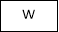Q x R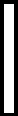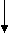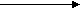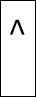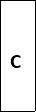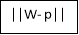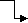P Q x 1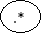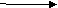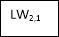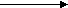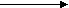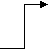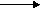R x 1 n a d c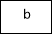Q x 1Q x 1 K x 1 K x 1

1.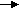K x QR Q x 1 Q KFigure 3: Network Structure

1) Input Layer: The input vector, denoted as p, is presented as the vertical bar in Figure 3. Its dimension is R × 1.

2) Radial Basis Layer: In Radial Basis Layer, the vector distances between input vector p and the weight vector made of each row of weight matrix W are calculated. Here, the vector distance is defined as the dot product between two vectors . Assume the dimension of W is Q×R. The dot product between p and the i-th row of W produces the i-th element of the distance vector ||W−p||, whose dimension is Q×1, as shown in Figure 3. The minus symbol, “” indicates that it is the distance between vectors. The transfer function in PNN has built into a distance criterion with respect to a center. In this paper, it is defined as

radbas (n) =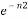Each element of n is substituted into Eq. 1 and produces corresponding element of a, the output vector of Radial Basis Layer. The i-th element of a can be represented as

Where Wi is the vector made of the i-th row of W and bi is the i-th element of bias vector b.

3) Some characteristics of Radial Basis Layer: The i-th element of a equals to 1 if the input p is identical to the ith row of input weight matrix W. A basis neuron which is radial along with a weight vector near to the input vector p will produce a value close to 1 and then its output weights in the competitive layer will pass their values to the competitive function. It is also possible that several elements of a are close to 1 since the input pattern is close to several training patterns.

4) Competitive Layer: There is no bias in Competitive layer. In Competitive Layer, the vector a is firstly multiplied with layer weight matrix M, producing an output vector d. The competitive function, denoted as C in Figure 3, produces 1 corresponding to the largest element of d, and 0’s elsewhere. The output vector of competitive function is denoted as c. The index of 1 in c is the number of numeral that the system can classify .

## V. EXPERIMENTAL RESULTS

The data set was divided into two separate data sets, the training data set and the testing data set. The training data set was used to train the network, whereas the testing data set was used to verify the accuracy and the effectiveness of the trained network for the classification of characters.

.The PNN was tested in more than 350 samples of telugu numerals and we have obtained an average recognition rate of 97%. Table 1 shows the performance of PNN.

 Number of Samples PNN 15 96.3% 50 96.8% 100 97.7% 125 97.6%

Table 1: Recognition Rate in Percentage

## VI. CONCLUSION

In this paper the PCA for feature extraction and the PNN for classification and recognition have been implemented. The performance of Probabilistic Neural Network classifier was calculated in the terms of classification accuracies and training performance. Probabilistic Neural Network gives fast and accurate classification and is a promising tool for classification of the characters.

### Recognition of Off-Line Handwritten Telugu Numerals Based On Principal Component Analysis

• Manubolu Sreenivasulu, Prasenjit Kumar Das

### Abstract

India is a multi-lingual multi-language country but there is not much work towards off-line handwritten character recognition of Indian languages . In this paper we have proposed principal component analysis (PCA) for feature extraction and used Probabilistic Neural Network (PNN) based model for classification and recognition of off-line handwritten numerals of telugu script. Principal component analysis is one of the important method for identifying patterns in data and expressing the data to highlight their similarities and differences. In high dimensional data it is difficult to identify patterns, where the luxury of graphical representation is not available, principal component analysis is a good method for analysing data. The performance of the Probabilistic Neural Network classifier was computed in terms of training performance and classification accuracies. Probabilistic Neural Network gives fast and accurate classification and is a promising model for classification of the characters .

Keywords – PCA, PNN, Classification and Recognition, Handwritten Characters

## I. INTRODUCTION

Character recognition (CR) is the important area in image processing and pattern recognition fields. The various applications of character recognition are includes library automation, banks, defense organizations, reading aid for the blind, post offices, language processing and multi-media design. Hence the research in character recognition is very popular. To recognize Hand-Written Characters (HWC) is an easy task for humans, but for a computer it is an extremely difficult job. This is mainly due to the vast differences or the impreciseness associated with handwritten patterns written by different individuals. Machine recognition involves the ability of a computer to receive input from sources such as paper and other documents, photographs, touch screens and other devices, which is an ongoing research area. Handwritten character recognition (HWCR) can be divided into two categories, namely, Offline Handwritten Character recognition where the image is sensed “off-line” from a part of a document and “Online” Handwritten Character recognition where the movements of the pen/tip can be recorded “on-line” as used in the pen based computer screen systems. Off-line recognition usually requires imperfect pre-processing techniques prior to feature extraction and recognition stages .

Telugu is the most popular script in India. It is the official language of the southern Indian state, Andhra Pradesh. The Telugu script is closely related to the Kannada script. Telugu is a syllabic language. Similar to most languages of India, each symbol in Telugu script represents a complete syllable. Officially, there are 10 numerals, 18 vowels, 36 consonants, and three dual symbols .

There are five major stages in the HCR problem: Image pre-processing, segmentation, feature extraction, training and recognition and post processing. Several works had been done on feature extraction for character recognition. The feature extraction method includes Template matching, Identification of similarities and differences, Histograms, Zoning, Graph description etc.

## II. DATA COLLECTION AND PREPROCESSING

Data collection for the experiment has been done from different individuals. Currently we are developing data set for Telugu. Earlier we had collected 250 Telugu numeral samples from 50 different writers. Writers were provided with a plain A4 sheet and each writer asked to write Telugu numerals from 0 to 9 at one time. Recently, we have again collected 150 Telugu numerals by 30 different writers. In this paper the data set size of 300 Telugu numerals is used. The database is totally unconstrained and has been created for validating the recognition system. The collected documents are scanned using the Canon-Lide scanner jet, which is usually a low noise and good quality image. The digitized images are stored as binary images in the BMP format. A sample of Telugu handwritten numerals from the data set is shown from figure 1.Figure 1: Handwritten Telugu Numeral Samples

Pre-processing:Figure 2: Pre-processing

Pre-processing includes the steps that are necessary to bring the input data into an acceptable form for feature extraction. The initial data is depending on the data acquisition type, is subjected to a number of preliminary processing stages. The pre-processing stage involves noise reduction, slant correction, size normalization and thinning .

For noise removal we used median filter. For better understanding the function of median filter, we added the salt and pepper noise artificially and removing it using median filter.

In character classification/recognition correcting the skew (baseline deviation from the horizontal direction) and the slant (average near-vertical strokes deviation from the vertical direction) is an important pre-processing step. The slant and slope are introduced by writing styles. Both corrections can reduce handwritten word shape variability and help the later operations such as feature extraction, classification and recognition .

Normalization is required as the size of the numeral varies from person to person and even with the same person from time to time. Thinning provides a reduction in data size; it extracts the shape information of the characters.

Thinning is the process of reducing the thickness of each line of pattern to just a single pixel . Here, we have used the morphology based thinning algorithm for better symbol representation. Thus, the reduced pattern is known as the skeleton and is close to the medial axis, which preserves the topology of the image. Figure 5 shows the steps involved in our method as far as pre-processing is considered.

## III. FEATURE EXTRACTION USING PRINCIPAL COMPONENT ANALYSIS

In this paper, the principal component analysis (PCA) is being used as an extraction algorithm of features. The PCA is a very successful techniques which has been utilized in image compression and recognition. The sole purpose of PCA is to decrease the big dimensions of data .

In this way we can identify patterns in data, and express the data in such a way as to present their differences and similarities. Because the patterns in data is hard to find in high dimension data, where the usefulness of graphical representation is not present, principal component analysis is a very good tool for analysing the data. The other advantages of PCA is that when you have found the patterns in data, and then you compress the data, i.e. by reducing the dimensions, with negligible loss of information .

#### Algorithm

Step 1: Get input as pre-processed image

Step 2: Subtract the mean

In order for the PCA to work correctly, it is needed for you to subtract the mean from each of the dimensions of data. The subtracted mean is the average in each dimension.

Step 3: Calculate the covariance matrix

The data is 2D, the covariance matrix will be 2×2.the definition for the covariance matrix for a set of data with n dimensional is:

Cn x n = (ci,j, ci,j = cov(Dimi,Dimj))

Step 4: Compute eigenvectors and eigenvalues of the covariance matrix

Because the covariance matrix is square, we are able to calculate the eigenvalues and eigenvectors for this matrix. The eigenvectors and eigenvalues will give us useful information about our data.

Step 5: Component choosing and creating a feature vector

In this step the notion of reduced dimensionality and data compression comes into it.When you look at the eigenvalues and eigenvectors from the previous section, you will be able to notice that the eigen values are quite not equal values. The fact is that the eigenvector with the highest eigenvalue is the main component of the data set. In fact the eigenvector with the larges eigenvalue was the one that pointed down the middle of the data. It is very important relationship between the dimensions of data. Generally, when the eigenvectors are found from the covariance matrix, the next step is to order the eigenvalue from highest to the lowest. This will give you the components in a very significant order.

Now it is required to form a feature vector, that is just a name for a matrix of vectors. It is constructed by taking the eigenvectors that you want to keep from the eigenvectors list, and creating a matrix with those eigenvectors in the columns.

Feature Vector = (eig1 eig2 eig3 ….. eign)

Step 6: Deriving the new data set

It is the last step in principle component analysist. When we have choosed the components or the eigenvectors, that we want to keep in our data and create a feature vector, we would take the transpose of that vector and will multiply it on the left of the true data set, transposed.

Final Data=Row Feature VectorXRow Data Adjust

Here the Row Feature Vector is the matrix of the eigenvectors in the columns transposed so that the eigenvectors will be in the rows, with the most important eigenvector at the top, and the Row Data Adjust will be z wiullthe mean-adjusted data transposed, ie. data items will be in each column, with each row holding a dimension which is separate.

## IV. CLASSIFICATION AND RECOGNITION USING PRABABILISTIC NEURAL NETWORK

Probabilistic neural networks (PNN) can be utilized for problems of classification. PNN is adopted for it has many advantages. Its training speed is many times faster than a BP network. PNN can approach a Bayes optimal result under certain easily met conditions . The most important advantage of PNN is that training is easy and instantaneous. Weights are not “trained” but assigned. Existing weights will never be alternated but only new vectors are inserted into weight matrices when training. So it can be used in real-time. Since the training and running procedure can be implemented by matrix manipulation, the speed of PNN is very fast.

The network classifies input vector into a particular class since that class has the highest probability to be correct. In this research paper, the PNN is of three layers: the Radial Basis Layer, the Input layer and the Competitive Layer. Radial Basis Layer calculates vector distances between input vector and row weight vectors in weight matrix. Those distances were scaled by Radial Basis Function not in a linear fashion. Then the Competitive Layer will find the shortest distance among them, and will then find the training pattern which is near to the input pattern depending on their distance. The network structure is illustrated in Figure 3. The symbols and notations are adopted as used in the book Neural Network Design . These symbols and notations are also used by MATLAB Neural Network Toolbox. Dimensions of arrays are marked under their names.

#### Input Radial Basis Layer Competitive LayerQ x RP Q x 1R x 1 n a d cQ x 1Q x 1 K x 1 K x 1

1.K x QR Q x 1 Q KFigure 3: Network Structure

1) Input Layer: The input vector, denoted as p, is presented as the vertical bar in Figure 3. Its dimension is R × 1.

2) Radial Basis Layer: In Radial Basis Layer, the vector distances between input vector p and the weight vector made of each row of weight matrix W are calculated. Here, the vector distance is defined as the dot product between two vectors . Assume the dimension of W is Q×R. The dot product between p and the i-th row of W produces the i-th element of the distance vector ||W−p||, whose dimension is Q×1, as shown in Figure 3. The minus symbol, “” indicates that it is the distance between vectors. The transfer function in PNN has built into a distance criterion with respect to a center. In this paper, it is defined as

radbas (n) =Each element of n is substituted into Eq. 1 and produces corresponding element of a, the output vector of Radial Basis Layer. The i-th element of a can be represented as

Where Wi is the vector made of the i-th row of W and bi is the i-th element of bias vector b.

3) Some characteristics of Radial Basis Layer: The i-th element of a equals to 1 if the input p is identical to the ith row of input weight matrix W. A basis neuron which is radial along with a weight vector near to the input vector p will produce a value close to 1 and then its output weights in the competitive layer will pass their values to the competitive function. It is also possible that several elements of a are close to 1 since the input pattern is close to several training patterns.

4) Competitive Layer: There is no bias in Competitive layer. In Competitive Layer, the vector a is firstly multiplied with layer weight matrix M, producing an output vector d. The competitive function, denoted as C in Figure 3, produces 1 corresponding to the largest element of d, and 0’s elsewhere. The output vector of competitive function is denoted as c. The index of 1 in c is the number of numeral that the system can classify .

## V. EXPERIMENTAL RESULTS

The data set was divided into two separate data sets, the training data set and the testing data set. The training data set was used to train the network, whereas the testing data set was used to verify the accuracy and the effectiveness of the trained network for the classification of characters.

.The PNN was tested in more than 350 samples of telugu numerals and we have obtained an average recognition rate of 97%. Table 1 shows the performance of PNN.

 Number of Samples PNN 15 96.3% 50 96.8% 100 97.7% 125 97.6%

Table 1: Recognition Rate in Percentage

## VI. CONCLUSION

In this paper the PCA for feature extraction and the PNN for classification and recognition have been implemented. The performance of Probabilistic Neural Network classifier was calculated in the terms of classification accuracies and training performance. Probabilistic Neural Network gives fast and accurate classification and is a promising tool for classification of the characters.

## REFERENCES

1. U. Pal1, T. Wakabayashi2, N. Sharma1 and F. Kimura2, “Handwritten Numeral Recognition of Six Popular Indian Scripts”,
2. Manubolu Sreenivasulu, Prasenjit Kumar Das, Mr. Rishi Mathur, “Numeral Recognition of Four Scripts: Bengali-Assamese, Gujarati, Oriya, Nepali”, First International Conference on Innovative Advancements in Engineering and Technology (IAET), 2014.
3. Panyam Narahari Sastry, Ramakrishnan Krishnan,“Isolated Telugu Palm Leaf Character Recognition Using Radon Transform – A Novel Approach”, 2012 World Congress on Information and Communication Technologies, 978-1-4673-4805-8/12/2012 IEEE.
4. Jian-xiong Dong and Dominique Ponson and Adam KrzyË™zak and Ching Y.Suen,“Cursive word skew/slant corrections based on Radon transform”.
5. Rafael C. Gonzalez, Richard E.woods and Steven L Eddins, “ Digital Image Processing using MATLAB”, Pearson Education, Dorling Kindersley,South Asia,2004
6. Lindsay I Smith,“A tutorial on Principal Components Analysis”, February 26, 2002.
7. D.F. Specht, “Probabilistic Neural Networks” Neural Networks, vol. 3, No.1, pp. 109-118, 1990.
8. M. T. Hagan, H. B. Demut, and M. H. Beale, Neural Network Design, 2002.
9. Mohd Fauzi Othman, Mohd Ariffanan Mohd Basri,“Probabilistic Neural Network For Brain Tumor Classification”, 2011 Second International Conference on Intelligent Systems, Modelling and Simulation.

## Related Services

View all

### DMCA / Removal Request

If you are the original writer of this essay and no longer wish to have your work published on the UKDiss.com website then please:

Related Services

Related Lectures